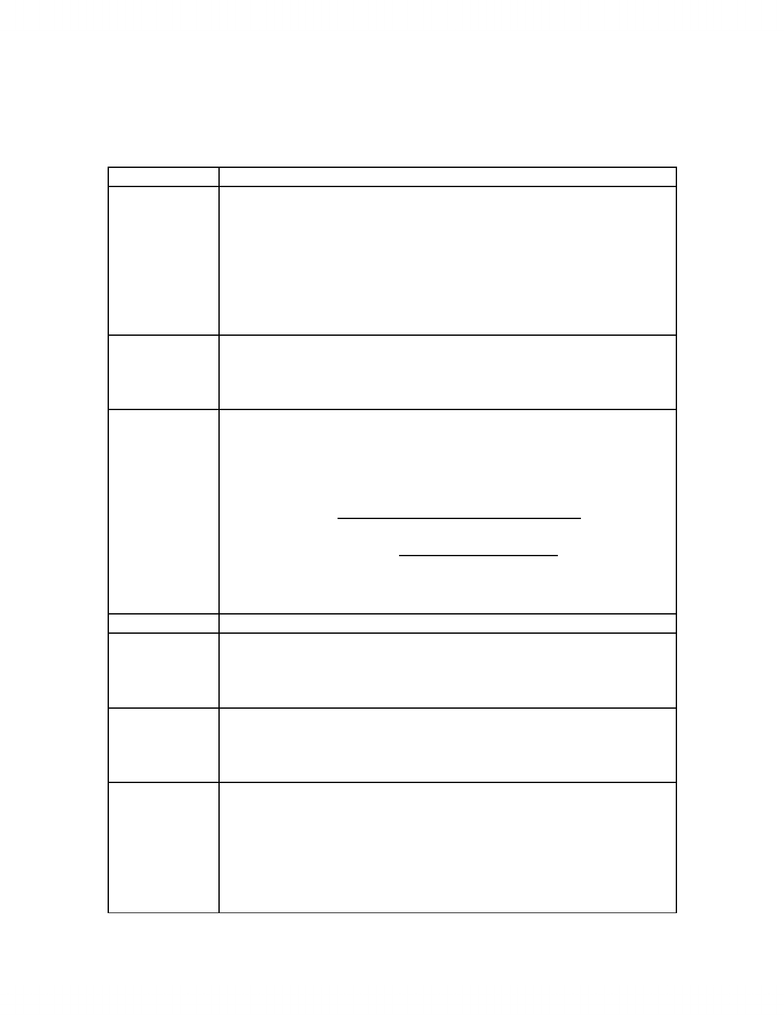# Notes taken during lecture

38 views3 pages
School
Department
Course
ProfessorN.B. ^ indicates superscript
LECTURE 2 – Chapters 3 & 5
Slide NumberNotes
23 -a mole = 6.022 x 10^23 units of something therefore sensible
-1 mol = 12 g of ^12 C
-mass to moles via dividing by g/mol
-moles to number of atoms via multiplying by Avogadro’s
number
-number of atoms to moles via dividing by Avogadro’s number
-moles to mass via multiplying by g/mol
24 -Ans: D
-formula” to determine number of molecules:
1g (1mol/____g)(6.022x10^23 molecules/1mol)
- fill in the blank with the MWs of the four options to determine D
25 -significance of empirical formulas: when presented with
unknown compound, one uses combustion analysis, which
releases elemental gases present in a certain ratio (thus
determining empirical formula)
-EX. M is an unknown metal
-Mass % = mass of element per mole of compound x 100%
Mass of 1 mole of compound
-therefore, 40.05% = 2 mol of O (15.999g/mol) x 100%
2 mol of O (15.999g/mol) + 1 mol of M (x)
-x is the molar mass of M
-solve for x, which is 47.90 g/mol -> Titanium
26 - balanced equation: 2NO + Ox -> 2NO2
27 EX. 1st step – write and balance equation
- 2 in front of H20 only
-100.0 g of CaC2 (1 mol/64.1g)(1 mol of HCCH/1 mol of CaCl2)
(26.036g/mol) = 40.62 g HCCH
28 -limiting reactant = limiting reagent = reactant that runs out first
-balanced equation: N2 + 3H2 -> 2NH3
-H2 is the limiting reagent BUT this assumes (incorrectly) that
there is no back reaction
29 -EX.
-42.7g Cr2O3 (1mol/151.99 g) = 0.281 mol Cr2O3
-9.8g Al (1 mol/26.98g) = 0.36 mol Al
-how much of Al needed to react all of Cr2O3 => 0.281 mol
Cr2O3 (2 mol of Al/ 1 mol of Cr2O3) = 0.562 mol of Al
-BUT do not have enough Al THEREFORE Al is limiting
reactant
www.notesolution.com
Unlock document

This preview shows page 1 of the document.
Unlock all 3 pages and 3 million more documents.

## Document Summary

Notes a mole = 6. 022 x 10^23 units of something therefore sensible for calculations about atomic-scale entities. 1 mol = 12 g of ^12 c. Mass to moles via dividing by g/mol. Moles to number of atoms via multiplying by avogadro"s. 29 number number of atoms to moles via dividing by avogadro"s number. Moles to mass via multiplying by g/mol. Fill in the blank with the mws of the four options to determine d. Formula to determine number of molecules: significance of empirical formulas: when presented with unknown compound, one uses combustion analysis, which releases elemental gases present in a certain ratio (thus determining empirical formula) Mass % = mass of element per mole of compound x 100% 2 mol of o (15. 999g/mol) + 1 mol of m (x) x is the molar mass of m solve for x, which is 47. 90 g/mol -> titanium therefore, 40. 05% = 2 mol of o (15. 999g/mol) x 100%

## Get access

\$8 USD/m\$10 USD/m
Billed \$96 USD annuallyHomework Help
Study Guides
Textbook Solutions
Class Notes
Textbook Notes
Booster Class